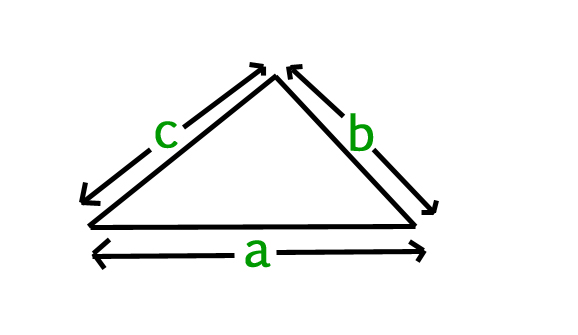Open in App
Not now

# Find Perimeter of a triangle

• Last Updated : 17 Feb, 2023

Given side (a, b, c) of a triangle, we have to find the perimeter of a triangle.

Perimeter :
Perimeter of a triangle is the sum of the length of side of a triangle.where a, b, c are length of side of a triangle.
Perimeter of a triangle can simply be evaluated using following formula :Examples :

```Input : a = 2.0, b = 3.0, c = 5.0
Output : 10.0

Input : a = 5.0, b = 6.0, c = 7.0
Output : 18.0```

## C++

 `// A simple C++ program to find the perimeter``// of triangle``#include ``using` `namespace` `std;`` ` `// Function to find perimeter``float` `findPerimeter(``float` `a, ``float` `b, ``float` `c)``{``     ` `    ``// Formula for finding a perimeter``    ``// of triangle``    ``return` `(a + b + c);``}`` ` `// Driver Code``int` `main() ``{``    ``float` `a = 2.0, b = 3.0, c = 5.0;``    ``cout << findPerimeter(a, b, c);  ``    ``return` `0;``}`` ` `// This code is contributed by Ankita saini`

## C

 `// A simple C program to find the perimeter``// of triangle``#include `` ` `// Function to find perimeter``float` `findPerimeter(``float` `a, ``float` `b, ``float` `c)``{``    ``// Formula for finding a perimeter of triangle``    ``return` `(a + b + c);``}`` ` `// Driver Code``int` `main()``{``    ``float` `a = 2.0, b = 3.0, c = 5.0;``    ``printf``(``"%f"``, findPerimeter(a, b, c));  ``    ``return` `0;``}`

## Java

 `// Java program to find perimeter``// of triangle``class` `Test {``    ``static` `float` `findPerimeter(``float` `a, ``float` `b, ``float` `c)``    ``{``        ``// Formula for Perimeter of triangle``        ``return` `(a + b + c);``    ``}`` ` `    ``// Driver method``    ``public` `static` `void` `main(String[] args)``    ``{``        ``float` `a = ``2.0``, b = ``3.0``, c = ``5.0``;``        ``System.out.println(findPerimeter(a, b, c));``    ``}``}`

## Python

 `# Python Program to find a perimeter``# of triangle`` ` `# Function to find perimeter``def` `findPerimeter(a, b, c):`` ` `    ``# Calculate the perimeter``    ``return` `(a ``+` `b ``+` `c)`` ` `# Driver Code    ``a ``=` `2.0``b ``=` `3.0``c ``=` `5.0``print``(findPerimeter(a, b, c))`

## C#

 `// C# program to find perimeter``// of triangle``using` `System;`` ` `class` `Test {``     ` `    ``static` `float` `findPerimeter(``float` `a, ``                           ``float` `b, ``float` `c)``    ``{``         ` `        ``// Formula for Perimeter of triangle``        ``return` `(a + b + c);``    ``}`` ` `    ``// Driver method``    ``public` `static` `void` `Main()``    ``{``        ``float` `a = 2.0f, b = 3.0f, c = 5.0f;``         ` `        ``Console.WriteLine(findPerimeter(a, b, c));``    ``}``}`` ` `//This code is contributed by vt_m. `

## PHP

 ``

## Javascript

 ``

Output :

`10.0`

Time Complexity: O(1)

Auxiliary Space: O(1)

My Personal Notes arrow_drop_up